Get instant live expert help with Excel or Google Sheets“My Excelchat expert helped me in less than 20 minutes, saving me what would have been 5 hours of work!”

#### Post your problem and you’ll get Expert help in seconds.

Your message must be at least 40 characters
Our professional Expert are available now. Your privacy is guaranteed.

# Average and ignore errors

We can use the AVERAGEIF or AGGREGATE function to find the average of a list of values while ignoring any errors that might exist in the data. The steps below will walk through the process.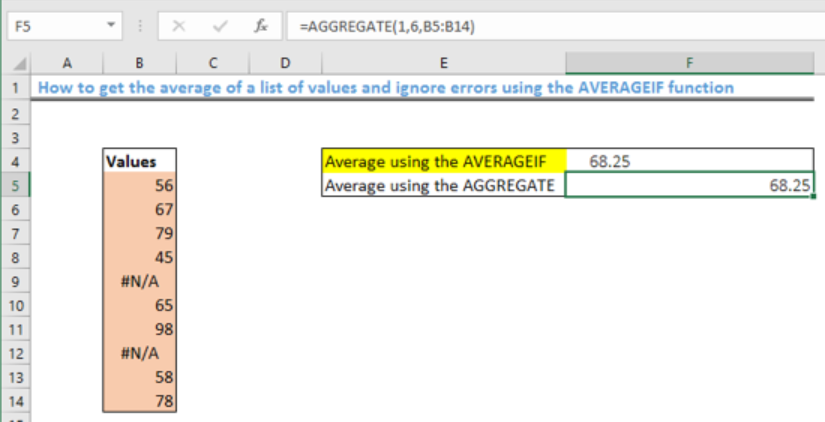Figure 1- Final result of the AVERAGEIF function

## General Formula

`=AVERAGEIF(values, “>=0”)`

## Formula

`=AVERAGEIF(B5:B14, “>=0”)`

## Setting up the Data

Our objective is to find the average of the list of values while ignoring all errors in the data.

• The list of values will be entered in the range B5:B14
• Cell F4 and F5 will contain the result after applying the AVERAGEIF function and AGGREGATE function respectively.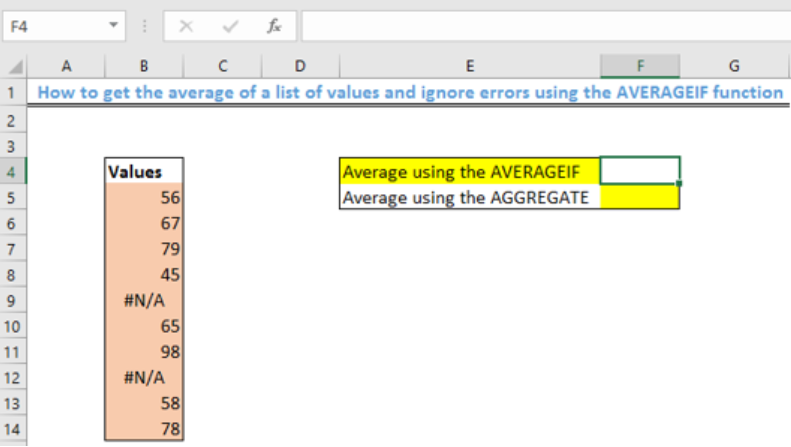Figure 2 – Setting up the Data

## Applying the AVERAGEIF function

• We will click on Cell F4
• We will insert the formula below into Cell F4
`=AVERAGEIF(B5:B14, “>=0”)`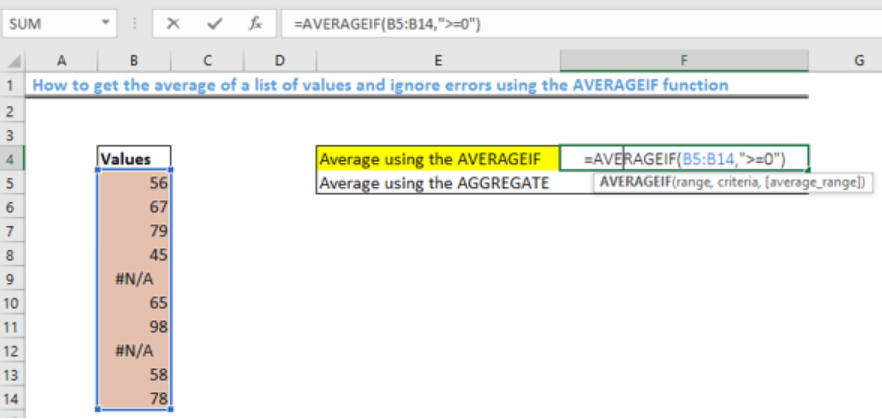Figure 3- How to use the AVERAGEIF function

• We will press the enter key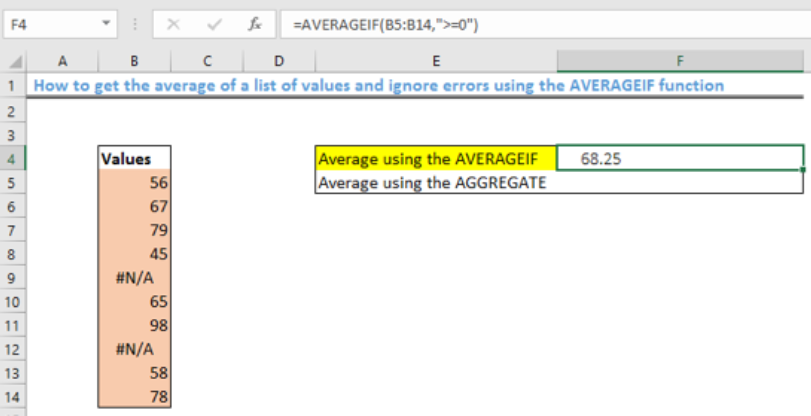Figure 4- Result of applying the AVERAGEIF function

## Using the Alternate AGGREGATE function

• We will click on Cell F5
• We will insert the formula below into Cell F5
`=AGGREGATE(1,6,B5:B14)`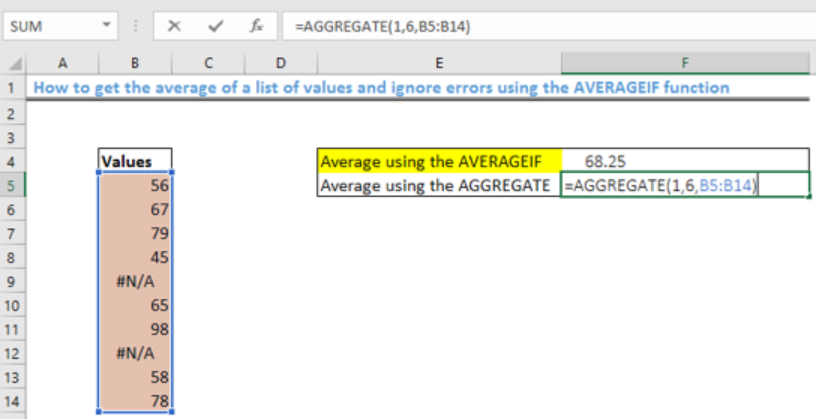Figure 5- How to use the AGGREGATE function

• We will press the enter key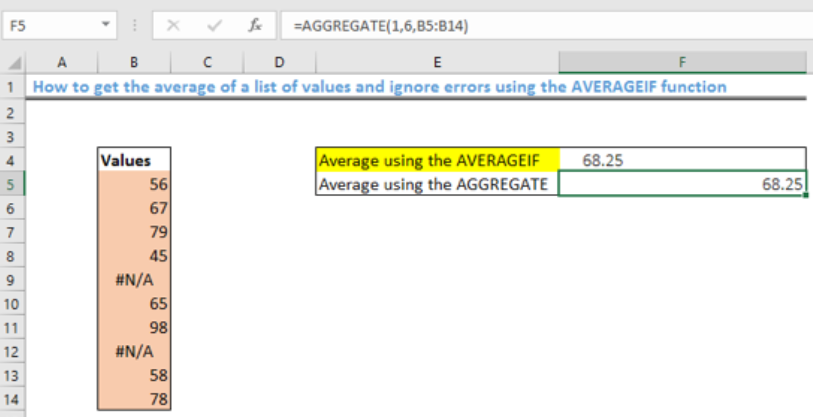Figure 6- Result of using the AGGREGATE function

## Explanation

• AVERAGEIF function

`=AVERAGEIF(values,">=0")`

The term “values” represents the range of the data: B5:B14. AVERAGEIF returns the average of the values that are greater than or equal to zero in the specified range, thus, filtering out any errors in the data.

• AGGREGATE function

`=AGGREGATE(1,6,values)`

“1” specifies average while “6” represents the option to ignore errors. Like the AVERAGEIF function, the AGGREGATE function also returns with the same answer.

## Instant Connection to an Expert through our Excelchat Service

Most of the time, the problem you will need to solve will be more complex than a simple application of a formula or function. If you want to save hours of research and frustration, try our live Excelchat service! Our Excel Experts are available 24/7 to answer any Excel question you may have. We guarantee a connection within 30 seconds and a customized solution within 20 minutes.

Are you still looking for help with the Average function? View our comprehensive round-up of Average function tutorials here.

### Did this post not answer your question? Get a solution from connecting with the expert.Another blog reader asked this question today on Excelchat:
Solution examplesI want to calculate an average of some values in a pivot table, however I cannot do that as some rows contain blanks.
Solved by I. C. in 58 minsI need help calculating average time elapsed. It is in dd:hh:mm:ss text format and so I can't calculate using =AVERAGEIF
Solved by O. E. in 11 minsHow do I calculate the average for a group of data that is referenced to multiple cells? I.e. If I wanted to know the average salary of 10 employees with the same salary grade but in different departments.
Solved by O. J. in 40 minsI contacted you yesterdaya bout how to use the =AVERAGE function, and for real numbers it works fine, but I tried to get the expert to explain to me how to use the =AVERAGE function when the cells have formulas in them that generate '0' value, so the AVERAGE function would ignore these cells in the averaging method, but got no answer. So, I need to know how use the =AVERAGE function so that when there are formulas in the selected cells to average, that EXCEL would ignore those cells like they have real numbers in them, which seems to work fine. Please advise...
Solved by C. J. in 30 minsI just need to edit this formula, {=IFERROR(AVERAGEIFS(B6:D6,F6:H6,"FALSE"),AVERAGEIF(B6:D6,"<>0"))}, to return "-" or "0" if there is nothing in column D. I can share the entire worksheet if need be.
Solved by V. A. in 47 mins## Subscribe to Excelchat.coAnother blog reader asked this question today on Excelchat: